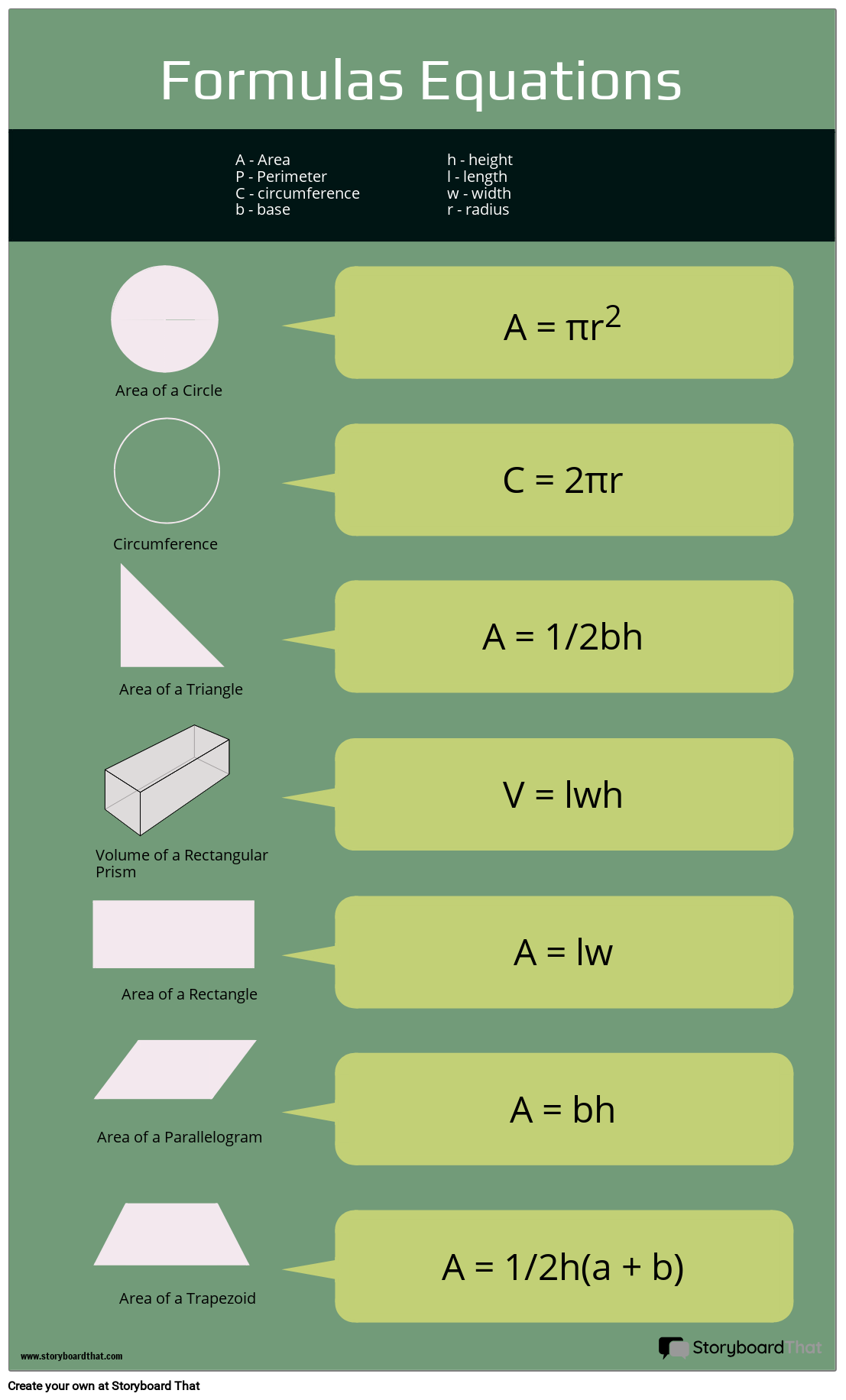# Equations &quot;Cheat Sheet&quot;#### Storyboard Description

Make equation cheat sheet infographics for students at the beginning or end of a unit!

#### Storyboard Text

• Formulas & Equations
• Area of a Triangle
• Circumference
• Area of a Circle
• A - Area P - Perimeter C - circumference b - base
• A = πr2
• C = 2πr
• A = 1/2bh
• V = lwh
• h - height l - length w - width r - radius
• Area of a Trapezoid
• Area of a Rectangle
• Volume of a Rectangular Prism
• Area of a Parallelogram
• A = lw
• A = bh
• A = 1/2h(a + b)
Over 30 Million Storyboards Created